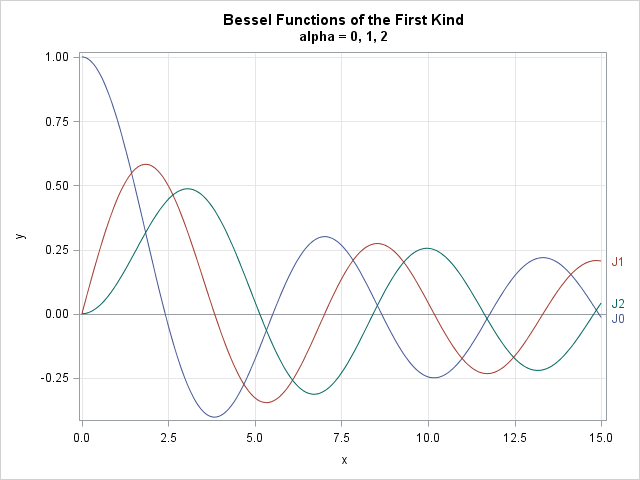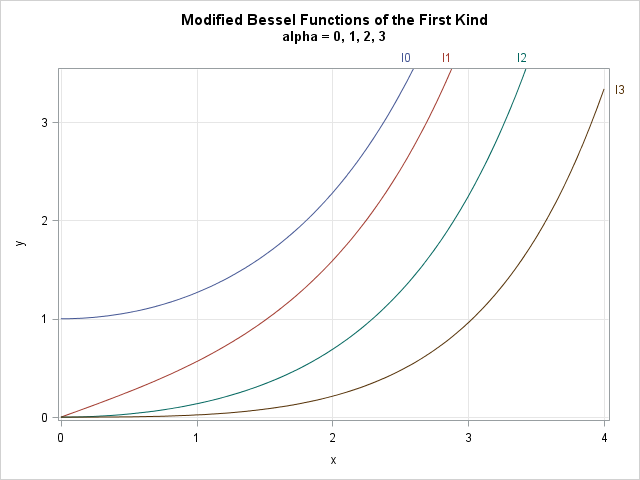When I was an undergraduate physic major, my favorite professor would start each class with a joke or pun. One day he began class with a paraphrase of a famous quote from the movie Star Trek 4: The Voyage Home (the one with the whales). "Today," my professor said, imitating Ensign Chekov's Russian accent, "I vill direct you to the nuclear Bessels!" (See the video at the end of this article.)

I've known for a long time that SAS software supports Bessel functions, which are used in physics to solve differential equations, but it was only recently that I started seeing examples of statistical distributions that involved Bessel functions as part of the formulas. (Do an internet search for "Bessel function" and "Bayesian inference.") Accordingly, I thought I'd mention that SAS has functions to compute Bessel functions. The JBESSEL function enables you to compute Bessel functions of the first kind. The IBESSEL function enables you to compute modified Bessel functions of the first kind. There are no built-in functions for computing the (less common) Bessel functions or modified Bessel function of the second or third kind.

The SAS/IML language also includes the JROOT function, which returns the roots of Bessel functions.

The family of Bessel functions includes a parameter, called the order of the Bessel function. The most important Bessel functions have orders that are small positive integers, which appear as subscripts. The following DATA step computes the values of J0, J1, and J2, which are Bessel functions the first kind of order 0, 1, and 2 (respectively) on the domain [0, 15]:

```data Bessel; do alpha = 0 to 2; name = cat("J", put(alpha, 1.)); do x = 0.0 to 15 by 0.05; J = jbessel(alpha, x); output; end; end; run;   title "Bessel Functions of the First Kind"; title2 "alpha = 0, 1, 2"; proc sgplot data=Bessel; refline 0 / axis=y; series x=x y=J / group=Name curvelabel curvelabelloc=outside curvelabelpos=auto; xaxis grid; yaxis grid label="y"; run;```You can see that the Bessel functions of the first kind look like damped sinusoidal functions, but the roots are not evenly spaced.

The modified Bessel functions of the first kind (or order α) are represented by the symbol Iα(x). The following DATA step evaluates Iα for α = 0, 1, 2, 3, on the domain [0, 4]:

```data ModBessel; do alpha = 0 to 3; name = cat("I", put(alpha, 1.)); do x = 0.0 to 4 by 0.05; I = ibessel(alpha, x, 0); output; end; end; run;   title "Modified Bessel Functions of the First Kind"; title2 "alpha = 0, 1, 2, 3"; proc sgplot data=ModBessel; series x=x y=I / group=Name curvelabel curvelabelloc=outside curvelabelpos=auto; xaxis grid; yaxis grid max=3.5 label="y"; run;```The modified Bessel functions grow exponentially. In some applications, the modified Bessel functions appear as part of the expression exp(x)Iα(x). In these applications, you can call the IBESSEL function as ibessel(alpha, x, 1), where the last argument specifies that the modified Bessel function should be multiplied by a decaying exponential function.

By the way, the German mathematician Friedrich Bessel (for whom Bessel functions are named) not only contributed to differential equations, but to astronomy and statistics. In astronomy, he was the first to use parallax to find the distance to celestial objects. In statistics, we remember Bessel every time that we compute a standard deviation. Bessel introduced the now-standard method of using n-1 in the denominator of the estimate of the sample variance. This is called "Bessel's correction," and it adjusts the estimate due to the fact that the sample mean is used as part of the computation of the sample variance.

If you've somehow missed seeing Star Trek 4: The Voyage Home, here is the clip where Chekov is trying to find the naval base "where they store the nuclear 'wessels'." The movie came out in 1986, which was before the fall of the Soviet Union.

Tags
ShareDistinguished Researcher in Computational Statistics

Rick Wicklin, PhD, is a distinguished researcher in computational statistics at SAS and is a principal developer of SAS/IML software. His areas of expertise include computational statistics, simulation, statistical graphics, and modern methods in statistical data analysis. Rick is author of the books Statistical Programming with SAS/IML Software and Simulating Data with SAS.

1.Chris Hunter on

Perhaps should have said:
Friedrich Bessel .... was the first to use parallax to find the distance to stellar objects.

Parallax was a key to estimates by Aristarchus and other ancient astronomers of the distince to the moon which is the closest celestial object.

•Interesting! Thanks for the correction. I guess the ancient astronomers used the diameter of the earth as the source of their parallax, whereas Bessel used the diameter of the Earth's orbit around the sun for his calculation.

2.What about BesselK function for non-integer order? It is a very important function which SAS does not have. Any idea how can we implement it?